Addition , written using the symbol $+$ , is the mathematical operation that represents the total number of objects together in a collection.

For simple addition problems, you can count dots to find the answer.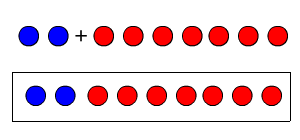$2+7=9$

There are $2$ blue dots and $7$ red dots. There are $9$ dots altogether.

To add numbers with more than one digit, you can visualize base ten blocks.

$115+37$

$115$ is equal to $100+10+5$ , so it can be represented by ONE $100$ block, ONE ten block, and FIVE unit blocks.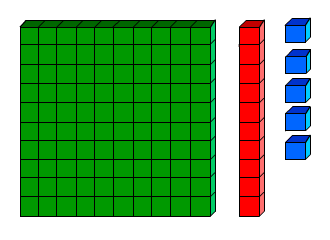$37$ is equal to $30+7$ , so it can be represented by THREE ten blocks and SEVEN unit blocks.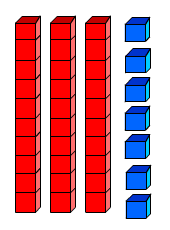To add, draw all the blocks together.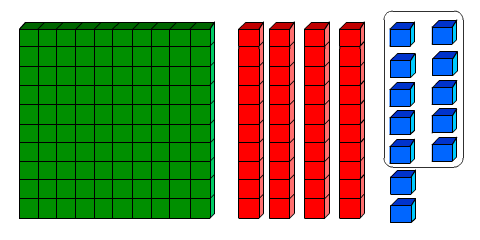Altogether we have $12=10+2$ unit blocks, so we can trade them up for ONE ten block and TWO unit blocks.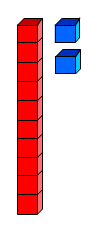Now we have $100+50+2$ or $152$ .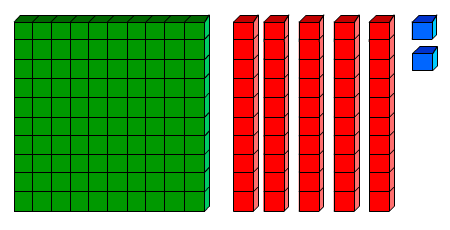So $115+37=152$ .

When you add by writing numbers vertically, and adding up the columns, you use "carrying," which is the same as the idea of trading up for the next-largest block.

$\begin{array}{l}\text{\hspace{0.17em}}\text{\hspace{0.17em}}\text{\hspace{0.17em}}5625\hfill \\ \underset{_}{+\text{\hspace{0.17em}}\text{\hspace{0.17em}}438}\hfill \end{array}$

First add the ones column.   $5+8=13$ , so write down the $3$ and carry the one.

$\begin{array}{l}\text{\hspace{0.17em}}\text{\hspace{0.17em}}\text{\hspace{0.17em}}5\text{\hspace{0.17em}}6\text{\hspace{0.17em}}2\text{\hspace{0.17em}}5\hfill \\ \underset{_}{+\text{\hspace{0.17em}}\text{\hspace{0.17em}}\text{\hspace{0.17em}}4\text{\hspace{0.17em}}3\text{\hspace{0.17em}}8\text{\hspace{0.17em}}\text{\hspace{0.17em}}}\hfill \\ \text{\hspace{0.17em}}\text{\hspace{0.17em}}\text{\hspace{0.17em}}\text{\hspace{0.17em}}\text{\hspace{0.17em}}\text{\hspace{0.17em}}\text{\hspace{0.17em}}\text{\hspace{0.17em}}\text{\hspace{0.17em}}\text{\hspace{0.17em}}\text{\hspace{0.17em}}\text{\hspace{0.17em}}\text{\hspace{0.17em}}\text{\hspace{0.17em}}3\hfill \end{array}$

Next add the tens column.   $1+2+3=6$ .

$\begin{array}{l}\text{\hspace{0.17em}}\text{\hspace{0.17em}}\text{\hspace{0.17em}}\text{\hspace{0.17em}}\text{\hspace{0.17em}}\text{\hspace{0.17em}}\text{\hspace{0.17em}}\text{\hspace{0.17em}}\text{\hspace{0.17em}}\text{\hspace{0.17em}}\text{\hspace{0.17em}}1\hfill \\ \text{\hspace{0.17em}}\text{\hspace{0.17em}}\text{\hspace{0.17em}}5\text{\hspace{0.17em}}6\text{\hspace{0.17em}}2\text{\hspace{0.17em}}5\hfill \\ \underset{_}{+\text{\hspace{0.17em}}\text{\hspace{0.17em}}\text{\hspace{0.17em}}4\text{\hspace{0.17em}}3\text{\hspace{0.17em}}8\text{\hspace{0.17em}}\text{\hspace{0.17em}}}\hfill \\ \text{\hspace{0.17em}}\text{\hspace{0.17em}}\text{\hspace{0.17em}}\text{\hspace{0.17em}}\text{\hspace{0.17em}}\text{\hspace{0.17em}}\text{\hspace{0.17em}}\text{\hspace{0.17em}}\text{\hspace{0.17em}}\text{\hspace{0.17em}}6\text{\hspace{0.17em}}3\hfill \end{array}$

Next add the hundreds column.   $6+4=10$ . Write down the $0$ and carry the $1$ .

$\begin{array}{l}\text{\hspace{0.17em}}\text{\hspace{0.17em}}1\text{\hspace{0.17em}}\text{\hspace{0.17em}}\text{\hspace{0.17em}}\text{\hspace{0.17em}}1\hfill \\ \text{\hspace{0.17em}}\text{\hspace{0.17em}}5\text{\hspace{0.17em}}6\text{\hspace{0.17em}}2\text{\hspace{0.17em}}5\hfill \\ \underset{_}{+\text{\hspace{0.17em}}\text{\hspace{0.17em}}4\text{\hspace{0.17em}}3\text{\hspace{0.17em}}8\text{\hspace{0.17em}}\text{\hspace{0.17em}}}\hfill \\ \text{\hspace{0.17em}}\text{\hspace{0.17em}}\text{\hspace{0.17em}}\text{\hspace{0.17em}}\text{\hspace{0.17em}}\text{\hspace{0.17em}}0\text{\hspace{0.17em}}6\text{\hspace{0.17em}}3\hfill \end{array}$

Next add the thousands column. $1+5=6$ .

$\begin{array}{l}\text{\hspace{0.17em}}\text{\hspace{0.17em}}1\text{\hspace{0.17em}}\text{\hspace{0.17em}}\text{\hspace{0.17em}}\text{\hspace{0.17em}}\text{\hspace{0.17em}}1\hfill \\ \text{\hspace{0.17em}}\text{\hspace{0.17em}}5\text{\hspace{0.17em}}6\text{\hspace{0.17em}}2\text{\hspace{0.17em}}5\hfill \\ \underset{_}{+\text{\hspace{0.17em}}\text{\hspace{0.17em}}4\text{\hspace{0.17em}}3\text{\hspace{0.17em}}8\text{\hspace{0.17em}}\text{\hspace{0.17em}}}\hfill \\ \text{\hspace{0.17em}}\text{\hspace{0.17em}}6\text{\hspace{0.17em}}0\text{\hspace{0.17em}}6\text{\hspace{0.17em}}3\hfill \end{array}$

For real numbers , the addition operation is commutative (that is, $a+b=b+a$ ; order does not matter) and associative (that is, $\left(a+b\right)+c=a+\left(b+c\right)$ ; grouping does not matter.) See Properties of Addition for more.